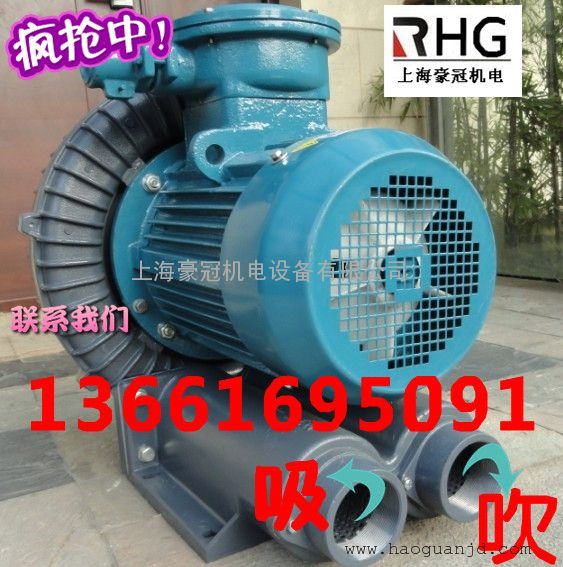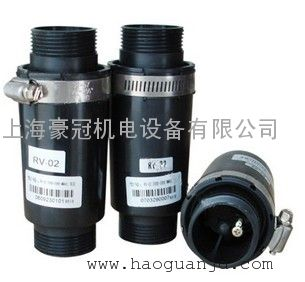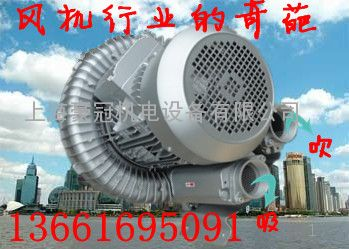# RHG vortex blower application note

RHG vortex blower application note
1. Air volume (Q):
The so-called air volume (also known as volume flow rate) refers to the flow velocity of the airflow through the cross-sectional area of â€‹â€‹the air duct. Generally, it is expressed by the following formula: Q=60VA
Q (air volume) = m3 / min
V (wind speed) = mc
A (cross-sectional area) = m2
2. Common conversion formula for pressure
1Pa=0.102mmAq
1mbar=10.197mmAq
1mmHg=13.6mmAq
1psi=703mmAq
1Torr=133.3pa
1 Torr = 1.333 mbar3. Common unit conversion table - air volume
1m3/min(CMM)=1000 l/min = 35.31 ft3/min(CFM)
4. Common noun description
(1) Standard state: 20 Â° C, absolute pressure 760 mmHg, relative humidity 65%. This state is abbreviated as STP, and generally the air weight of 1 m3 is 1.2 kg in this state.
(2) Absolute pressure of air: the sum of the atmospheric pressure displayed by the local atmospheric pressure gauge plus the gauge pressure, generally expressed in kgf/m2 or mmaq.
(3) Reference state: 0 Â° C, absolute pressure 760 mmHg, relative humidity 0%. This state is abbreviated as NTP. Generally, the air weight of 1m3 is 1.293kg in this state.
5. Pressure
(1) Static pressure (Ps): The so-called static pressure is the force that the fluid is applied to the surface of the appliance and perpendicular to the surface. Generally, it is caused by gravity and the push of the fan in the fan. It is often used in kgf/m2 or mmaq. Said, and can be directly obtained through measurement. In the air duct of the fan, the static pressure value in any direction is constant and there are positive and negative points. If the static pressure value is positive, the air duct is currently being inflated, and if the static pressure value is negative, the air duct is It is currently being squeezed.
(2) Dynamic pressure (Pv): The so-called dynamic pressure is the pressure formed by the velocity of the fluid flowing in the air duct. It is often expressed in kgf/m2 or mmaq.
(3) Full pressure (PT): The so-called full pressure is the sum of static pressure and dynamic pressure, and is often expressed in kgf/m2 or mmaq. The total pressure value in the fan is fixed and will not change due to the shrinkage of the duct.
6. Wind pressure and temperature changes will affect the density of the air. Therefore, under other conditions, when the temperature changes, the wind pressure must be corrected according to the following relationship to obtain the wind pressure value under standard conditions:
P = P'[(273 + t)/293] (mm Aq)
Similarly, when the air density changes, the wind pressure value can be corrected as follows:
P = P'(1.2/Î³ ) (mm Aq)
In the formula, the value on the right side of the equal sign is the measured pressure, temperature and air density of P', t, Î³, and the like.
7. The relationship between pressure and speed is how fast the pressure is fixed. It is impossible to change the speed without changing the pressure. Similarly, it is impossible for the pressure to change at the same speed.
Pv=r Ã— (V2 / 2g)
Pv: dynamic pressure (mmAq) r: specific gravity of air (kg/m3)
g: gravitational acceleration (m/s2) = 9.8 V: wind speed (m/s
RHG vortex blower application note